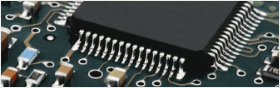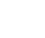• Home
• Instant Online Quote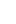• PCB Assembly
• PCB Capabilities
• Feedback
• Resources• About Us#### Right Angle and Differential Traces, Serpentine Lines in PCB Layout2018/12/25 10:01:42

Layout is one of the most basic work skills of PCB design engineers. The quality of the trace will directly affect the performance of the entire system. Most high-speed design theories will eventually be implemented and verified by Layout. It can be seen that the wiring is crucial in high-speed PCB design. The following will analyze the rationality of some situations that may be encountered in actual wiring, and give some more optimized routing strategies.

Right Angle

Right angle tracks are mostly often needed during PCB design and are almost one of the standard for measuring cable quality.

Can you guess how much impact does a trace with the right angle will have on the signal transmission? In general, a right-angled stroke will change the track width of the transmission lines, which will cause the impedance to be not consistent.as a matter of fact, not only the line of the right angle, but also the angle and the sharp line will cause the impedance change accordingly.

The effect of the right-angled line on the signal is mainly reflected in three aspects:
First, the corner can be equivalent to the capacitive load on the transmission line, slowing down the rise time;
Second, the impedance discontinuity will cause signal reflection;

The third is the EMI generated by the right angle tip.The parasitic capacitance from the right angle of the transmission line can be calculated by the following empirical formula:C=61W(Er)[size=1]1/2[/size]/Z0

With the given format, C refers to the equivalent capacitance of the corner (unit: pF), and W is the width of the trace (unit: inch), εr is to the dielectric constant of the medium, Z0 is the characteristic impedance from the transmission line. Take it as a example, for a 4Mils 50 ohm transmission line (εr is 4.3), the capacitance at a right angle is about 0.0101 pF, so in turn it can estimate the resulting rise time variation: T10-90%=2.2*C*Z0/2 = 2.2*0.0101*50/2 = 0.556ps

And we can see from the calculation that the capacitance effect brought by the right angle trace is very small. As the line width of the right-angled trace increases, the impedance at that point will decrease, and a certain signal reflection phenomenon will occur. We can calculate the equivalent impedance after the line width is increased according to the impedance calculation formula mentioned in the transmission line section, and then Calculate the reflection coefficient according to the empirical formula:

ρ=(Zs-Z0)/(Zs+Z0).

Normally, the impedance change caused by a right-angled trace is between 8% and 19%, so the reflection coefficient is at most about 0.1. What is more, as can be seen from the figure below, the transmission line impedance changes to the minimum during the W/2 line length, and then returns to the normal impedance after W/2 time. The entire impedance change time is extremely short, often at 10 ps. Inside, such fast and small changes are almost negligible for general signal transmission.Many people have such an understanding of right-angled lines. They believe that the tip is easy to emit or receive electromagnetic waves and generate EMI. This has become one of the reasons why many people think that they cannot be crossed at right angles. However, the results of many actual tests show that right-angled traces do not produce significant EMI than straight lines. Perhaps the current instrument performance, test level limits the accuracy of the test, but at least illustrates a problem, the radiation of the right angle trace is less than the measurement error of the instrument itself.

In a general level, right-angled lines are not as bad as you might think. At least in GHz applications, any impact such as capacitance, reflection, and EMI are almost will not to show in TDR test. The concentrate of high-speed PCB design engineers normally are on layout, power or ground design, or routing designing.  And others such as vias, although the impact of the right-angled line is not very bad, it not meaning that we can go right-angle free, paying much attention to the details in the design is a very basic quality of each professional engineer. Moreover, with the fast development of digital circuits boards, the frequency of signals handled by engineers will still to be increased in near future.##### You might likeSebastien

1

• Following

0

• Followers

0

PCB Prototype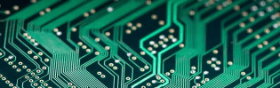PCB Instant Quote

x mm

Quantity

Quote Now

PCB Assembly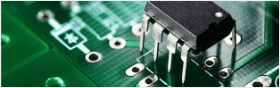SMT-StencilComponents Sourcing